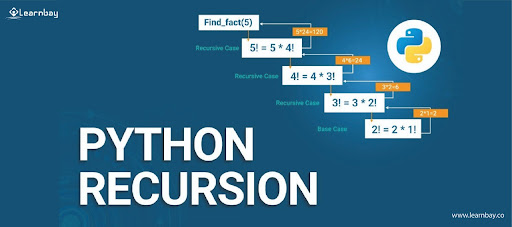# Basics of Functions In Python - A Glance

By Vineeth Kumar Published in Python 9-10 mins

Transforming the data in an effective way and then utilizing them in an optimized manner while programming is known as Functions in python. Also, executing the block only when it is called is known as a function.

With the help of 'functions' programs, you can break into smaller chunks of code. When you need to write the same code several times you can use that code in a function and call that function whenever you want to execute that code. In this article let me take you through different functions used in python along with some of the examples.

## Defining a function

A group of reusable code that is called anywhere in a program eliminates the need for writing the same code repeatedly. A function helps programmers for writing modular codes. When you write a function inside a class, it becomes a method. After the function is defined we can use it by calling the function 'fun()'.

A function is used to provide the necessary functionality. Simple rules for defining a function are as follows:

• The 'def' keyword is used to begin a function, which is then followed by the name and parentheses'()'.
• You can input any parameters or arguments within the parentheses.
• For documentation, the first function statement can be made optional or docstring.
• Every function having a code block starts with a colon (:) which is indented.
• The return(expression) statement retains a function by providing the caller with a returned expression.

Example: Defining a function:

| # A simple python function

def fun():

print("Hello Data Science")

Example: Calling a function:

Once functions are created you can call them by using the name of that function which is followed by a parenthesis that contains parameters for that particular function.

| # A simple python function

def fun():

print("Hello Data Science")

# Driver code to call a function

fun()

Example:

Depicting the first call to the function and second call to the same function.

def my_function(Fun):
print(Fun + "Classes")

my_function("Hello")
my_function("Data")
my_function("Science")

Output
Hello Classes
Data Classes
Science Classes

## What are the various types of functions in python?

In python, you have two important types of functions

• Built-in functions
• User-defined functions

### Built-in functions

Default functions which are already programmed in python software called 'Built -in function'. You have a wide range of built-in functions available in python. Some of the built-in functions are

• int
• list
• tuple
• float
• string
• set
• bin
• oct
• hex
• dictionary

### User-defined function

The functions specifically you write to define certain tasks fall under user-defined functions. It can also be a library for someone else. If you are working on a larger project you can break the workload by maintaining different functions.

Example:

# program for user-defined functions

def multiply_num(x,y):
mul = x*y
return mul

num1 = 5
num2 = 6
print("The Product of: ", multiply_num(num1,num2)

The Product of: 30### Recursive Functions in Python

#### What do you mean by recursion in python?

The process of defining anything by itself is known as recursion. A function has the ability to call other functions. Retrieving the own function is possible by using a function.

Example:

| # To find the factorial number
def calc_fact(x):
if x == 1:
return 1
else:
return(x * calc_fact(x-1))
num = 4
print ("Factorial of", num, "is", calc_fact(num))

In the above example, calc_fact() is a recursive function in python as it calls the function by itself. The function call multiples with the factorial number 1 until it is equal to 1.

Note : If a recursive function does not provide a base condition that ends the recursion, the function will call itself, indefinitely.

### Lamda Function

#### What are Lambda Functions in python?

Lambda Functions in python are also known as anonymous functions which means the function is without a name. As you use the 'def' keyword for normal function in python similarly, the Anonymous function uses the lambda keyword to define the function in python.

The syntax for lambda = lambda arguments: expression

• Any number of arguments can be there in this function however only one expression gets evaluated and returned.
• If the function objects are required you can use Lambda functions.
• Grammatical arrangements are minimized to a single expression in lambda functions.
• lambda has many uses apart from other types of expressions in a particular area of programming.

Example:

string = 'HellodataScience'
#Lambda returns a function object
print(lambda string : string)

Output
<function at 0x0000016282865820 >

## Take away

As a programmer a precise and optimal way of writing code would help better rather than having complications. Functions would be a better option as you can break the program into smaller programs.

If you're interested in learning how to use python effectively, check out the data science course with special python help from an industrial specialist and best-in-class resources. If you're just getting started with Python follow us on Facebook, Youtube, Linkedin, Twitter.

Tags

#Functions in python#types of function#lambda functions#functions in python example#types of functions in python#user defined functions in python#python functions list#main function in python#built in functions in python#define function in python#calling a function in python#user-defined functions in python#lambda functions python#python list of lambda functions#what is lambda function in python with example